Happy Holiday! Key mailing dates for package to arrive by Dec 22 within Canada is Dec 12 for Canada Post Expedited Parcel.

# Soil Moisture Sensor Module (Capacitive) Guide

Posted by Kelly M on

The Soil Moisture Sensor Module (Capacitive) acts like a capacitor where the water level changes the capacity and we can measure that charge and discharge timing. It is a popular module for measuring the moistness of soil. The biggest advantage over a traditional moisture sensor is that the capacitive sensor doesn't corrode.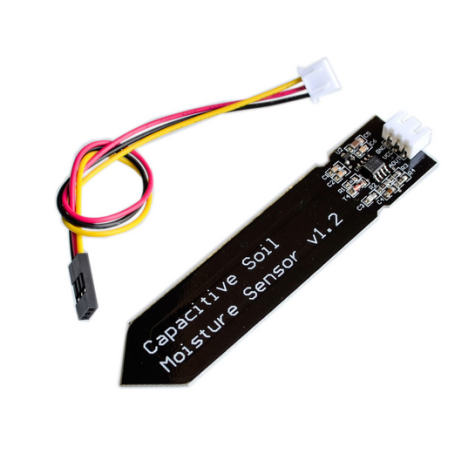In this article, we will talk about how to wire and set up the module for operation with an Arduino Board.

### Wiring

Most soil moisture sensor module operates between 3.3V to 5V and produce an analog output. For this project, we will be using Soil Moisture Sensor Module (Capacitive), the one we carry in our shop.

## Wiring Guide

Connect the pins the female to female jumper wires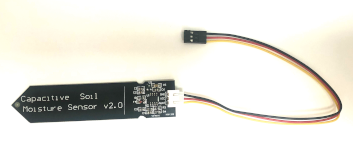Then, connect the jumper wires with male to male jumper wires for connecting Arduino Board.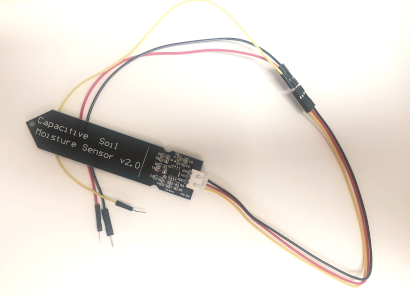• Module GND -- Arduino GND pin
• Module VCC -- Arduino VCC pin
• Module AUOT -- Arduino A0 pin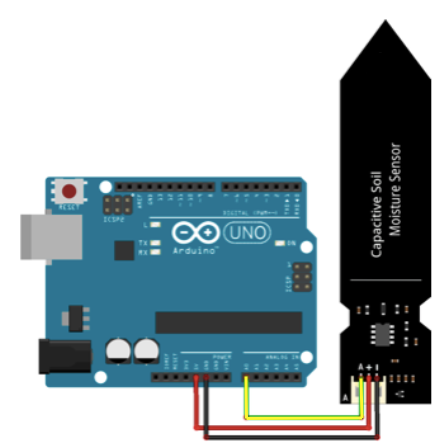## Programming

Before we get the accurate value for result, we need to know what are the values when the sensor is in water and when it is dry. If you want to skip the steps and jump to the Final Build

Step 1: Create a variable for storing the sensor's output

`int value = 0;`

Step 2: Set up serial communication between Arduino and the sensor.

```void setup(){
Serial.begin(9600);
}```

Step 3: Read the sensor's output

```void loop(){

Step 4: Print out the value and wait for half a second for the next reading.

```  Serial.println(value);
delay(500);
}```

### Full Code

```int value = 0;

void setup(){
Serial.begin(9600);
}

void loop(){
Serial.println(value);
delay(500);
}
```

Now that you can measure the threshold values for the sensor. The values will vary depending on the water level.

Step 1: Put the sensor in a dry spot and record the highest value. I got 586 in this example.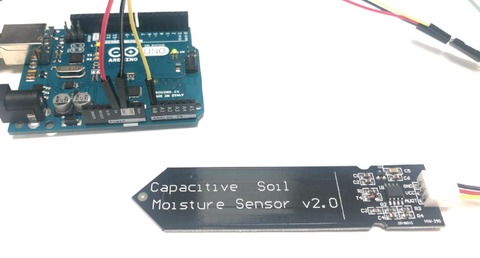Serial Monitor: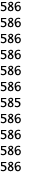Step 2: Put the sensor in a cup of water and record the lowest value. I got 267 in this example.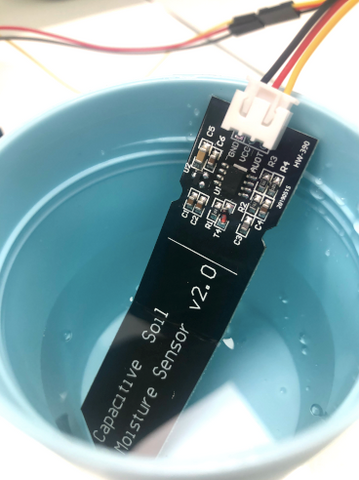Serial Monitor:Step 3: Wipe down the water on the sensor.

## Final Build

Now that we know the values when the sensor is in water and when it is not. We can use those values as the threshold and determine the moistness percentage with the map function on Arduino. If you want to skip the steps and jump to the Full Code

Step 1: Create variables to store the threshold values, sensor's output and percentage result.

```const int dry = 586;
const int wet = 267;
int value = 0;
int percentage = 0;```

Step 2: Set up serial communication between Arduino and the sensor.

```void setup(){
Serial.begin(9600);
}```

Step 3: Read the sensor's output.

```void loop(){

Step 4: Use map function to change the range from the two threshold values to be from 0 to 100.

`percentage = map(value, dry, wet, 0, 100);`

Step 5: Print message according to the percentage, and wait for half a second for next reading.

```if (percentage >= 100){
Serial.println("100%");
}
else if (percentage <= 0){
Serial.println("0%");
}
else {
Serial.print(percentage);
Serial.println("%");
}
delay(500);
}```

### Full Code

```const int dry = 586;
const int wet = 267;
int value = 0;
int percentage = 0;

void setup(){
Serial.begin(9600);
}

void loop(){
percentage = map(value, dry, wet, 0, 100);
if (percentage >= 100){
Serial.println("100%");
}
else if (percentage <= 0){
Serial.println("0%");
}
else {
Serial.print(percentage);
Serial.println("%");
}
delay(500);
}
```

### Output

When it is in the water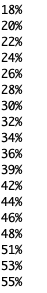When it is not in water